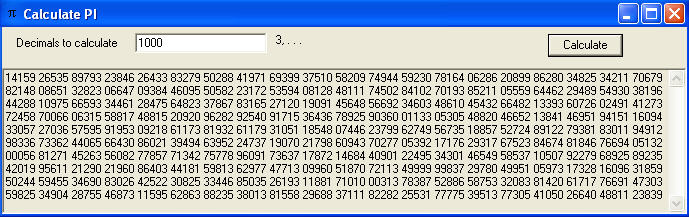Calculate PI using VB.NET 2008

Here is a simple program written in VB.NET 2008 to calculate as many decimal places of letter Pi (the ratio of the circumference to the diameter of a circle) as necessary.

Screenshot: